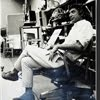## Eugene Ray SDSU 1970'sEugene Ray 1970's. photo credit: Tom Davis

## Sunday, July 13, 2014

### TESLA OUR FRIEND / architecture as situation / environ as energy

+"THE FANTASTIC INVENTIONS+
+OF NIKOLA TESLA"+ (1993)
^^^^^^^^^^^^^^^^^^
>i purchased yesterday from my<
>bookseller friend dennis wills<
+(D.G. WILLS BOOKS+
+LA JOLLA, CALIF)+
>is a special & profound read< !
^^^^^^^^^^^^^^^^^
+DAVID HATCHER CHILDRESS+
>the author, made famous by his many books<
>but more recently by his starring role in the<
+"ANCIENT ALIENS"+
>television series on the history channel 2<
>i had a discussion w/ at the "open minds"
>ufo conference at fort mcdowell arizona<
^^^^^^^^^^^^^^^^^^^^^^^^
+I POINTED OUT THE WORK+
>of the hong kong scholar<
+WUN CHOK BONG IN HIS+
>(ancient biotronics history)<
+"THE GODS MACHINES"+
^^^^^^^^^^^^^^^^^^^
>i photographed the tesla book next to the<
+(CHAPTER 9 / "TESLA &+
+MARTIAN PYRAMIDS")+
>childress links tesla w/ ufo activity via<
>a marconi secret latin america factory<
^^^^^^^^^^^^^^^^^^^^^^^^
>tesla's 1928 transcendental manifestation<
+(NEXT STEP, UFO'S)+ !
>a vertical take off helicopter type aircraft<
^^^^^^^^^^^^^^^^^^^^^^^^^
>these patent drawings of teslas amazing<
>design for a vertical take off aircraft<
+(TESLA & LEONARDO+
>remind us of leonardo's 16c vision<
^^^^^^^^^^^^^^^^^^^^^^
>in 1967 i built a radiant new orleans abode<
>for the likes of nikola tesla w/ photos by<
+(AN ABODE FOR+
+NIKOLA TESLA)+
>my friend and l.s.u. alum john messina<
^^^^^^^^^^^^^^^^^^^^^^^^
>at sdsu i designed and taught a class in<
>building transcendental manifestations<
+(COLOR-LIGHT-ENERGY+
+SYNERGETIC ENVIRON)+
^^^^^^^^^^^^^^^^^^^^^^^^
>tesla's "death ray" invention caused such<
>community concern as to cause the<
+(PUBLIC SIMPLETONS+
+DID NOT SUPPORT)+ !
>dismantling of his biotronic tower<
^^^^^^^^^^^^^^^^^^^^^
>a van de graff electrostatic generator of<
>the carnegie institution created a flow<
+(TESLA'S ENERGY VISION+
+LED to the A.C. CURRENT)+
>of radiant energy similar to teslas<
^^^^^^^^^^^^^^^^^^^^^^
>transcendent device for photographing the<
+(DAVE FOBES : / HAS HIS+
>(is this a good idea)< ?!!!
+THOUGHTS RECORDED)+
>thought images of visionary artist-seers<
^^^^^^^^^^^^^^^^^^^^^^^^^^
>four centuries preceding tesla's transcendent<
>design vision, leonardo de vinci had an even<
+(FORTRESS W/ COMPOUND+
+CURVED OUTER WALLS)+
>greater flow of innovative visionary work<
^^^^^^^^^^^^^^^^^^^^^^^^
>the electro-magnetic nature of the human<
>body causes a powerful reaction to our<
+w/ HUMAN CHACRAS)+
>inter action w/ tphysical environment<
^^^^^^^^^^^^^^^^^^^^^^^^
>our synergetics biotronic work at sdsu<
>was done before i knew of the radiant<
+BECAME OUR PASSION)+
>oeuvre of tesla & leonardo da vinci<
^^^^^^^^^^^^^^^^^^^^^^
>at sdsu our mathematics scholar friend ron<
>schaefer was our expert in verification of<
+(MY CONICAL-SPHERICAL+
>the perfect volume for strong biotronics<
^^^^^^^^^^^^^^^^^^^^^^^^
>as early as 1972 at sdsu, i constructed a<
>slide presentation titled "architecture as<
+(UPPER RIGHT IMAGE IS+
+my idec SLIDE LECTURE)+
>situation / environment as light" (idec)<
^^^^^^^^^^^^^^^^^^^^^^^^
+ARCHIVAL NOTE+
>i have donated my slide lectures to<
>the sdsu library archive but it still<
+(MAYBE THNOC+
+COULD HELP)+ !
>awaits funding for sdsu digitizing<
^^^^^^^^^^^^^^^^^^^^
+MY VIDEOS AVAILABLE+
>(i am forever grateful<
>to prof. robert ray)< !
+on the WWW INTERNET+
^^^^^^^^^^^^^^^^^^
eugene ray, mfa, architect
professor emeritus, sdsu